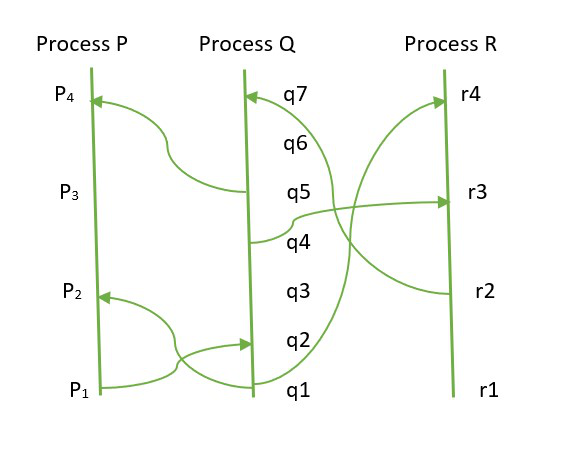# Event Ordering in Distributed System

• Difficulty Level : Expert
• Last Updated : 04 Mar, 2022

In this article, we will look at how we can analyze the ordering of events in a distributed system. As we know a distributed system is a collection of processes that are separated in space and which can communicate with each other only by exchanging messages this could be processed on separate computers or it could even be multiple processes within the same computer.

A defining characteristic is that the delay of transmitting a message is not negligible compared to the time between events in a single process. There is a fundamental limitation when it comes to ordering events in a distributed system and that is sometimes it is simply not possible to tell if one event occurred before another one if you take the complete set of events within a distributed system.

We can only at best define a partial ordering within those events or an intuitive understanding of the ordering of events is naturally associated with the time at which events occur.

However, in a distributed system we cannot count on physical clocks even if the computers themselves have physical clocks, because two clocks on two different systems can never be perfectly synchronized they will always, in reality, be some drift between any two physical clocks.

So, mostly physical time is ignored when it comes to ordering events within a distributed system, events are only observable within a system.

Now observable events are primarily sending and receiving of messages.

We are assuming that a single process is completely sequential in that we can take all the events within a single process and totally order them. So that we get the exact sequence in which those events occurred within that process.

• An event is simply the sending or receiving of a message.
• Now, partial ordering of events can be denoted by “⇢”, and we can define it as if – we have two events and be within the same process and a comes before b then a ⇢ b i.e.; a happened before b.
• Now, if two processes are communicating with each other a is the sending of a message and b is the receipt of that message by another process then we define an as happening before b.
• Lastly, we have a transitive property which says if a happened before b and b happened before c then a happened before c as well, a⇢b and b⇢ c then a⇢ c.
• Since this is a partial ordering it could very well happen that for two events a and b neither happened before the other we simply cannot tell by looking at the observable events in the system and in that case we call a and b to have happened concurrently.• P, Q, R are the process and each dot in the line denotes the events, the curve lines denote messages being sent between processes from this representation we can see relate p1 and r4 and we can say that p1 happened before r4 because p1 sent a message to Q and that was event q2 we move forward in time within the process Q we send a message to process R which was received over here at r3. So, we can say p1 happens before r4. and r2, q6 are concurrent as they cannot causally affect one another.
• Happened before relation is an irreflexive partial ordering on the set of all events happening in the system i.e.; (a⇢ a) is not true for any event a.
• This relates back to Einstein’s general theory of relativity where events are ordered in terms of messages that could possibly be sent.

### Logical Clocks:

A logical clock is a way of assigning a number to an event where that number can be thought of as the time at which the event occurred and the notation we use here is Ci<a> of an event a, where C is used to denote Clock. These numbers are purely logical they do not have any relationship to physical time

### Conditions For logical clocks:

• If a happens before b the logical time of a should be lesser than the logical time of b of a process Pi
• a⇢ b then C<a><C<b>
• If a is the sending message by process Pi and b is the receipt of that message by Pj the
• Ci<a><Cj<b>.
• The logical clock is associated with timestamps.

So given these conditions, We define a clock function within a process by simply incrementing the process’s own clock as we go from event to event i.e.; Each process Pi increments Ci between any two successive events.

So, now we have a partial ordering of the events within a distributed system and we have a way to assign numbers to those events such that we can construct logical clocks out of them.

My Personal Notes arrow_drop_up
Recommended Articles
Page :